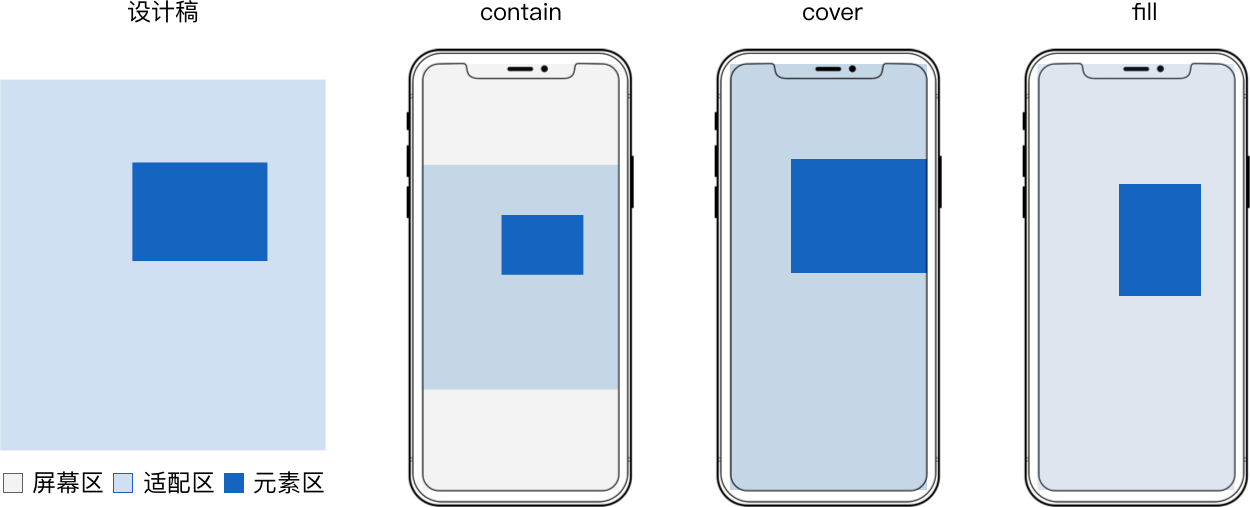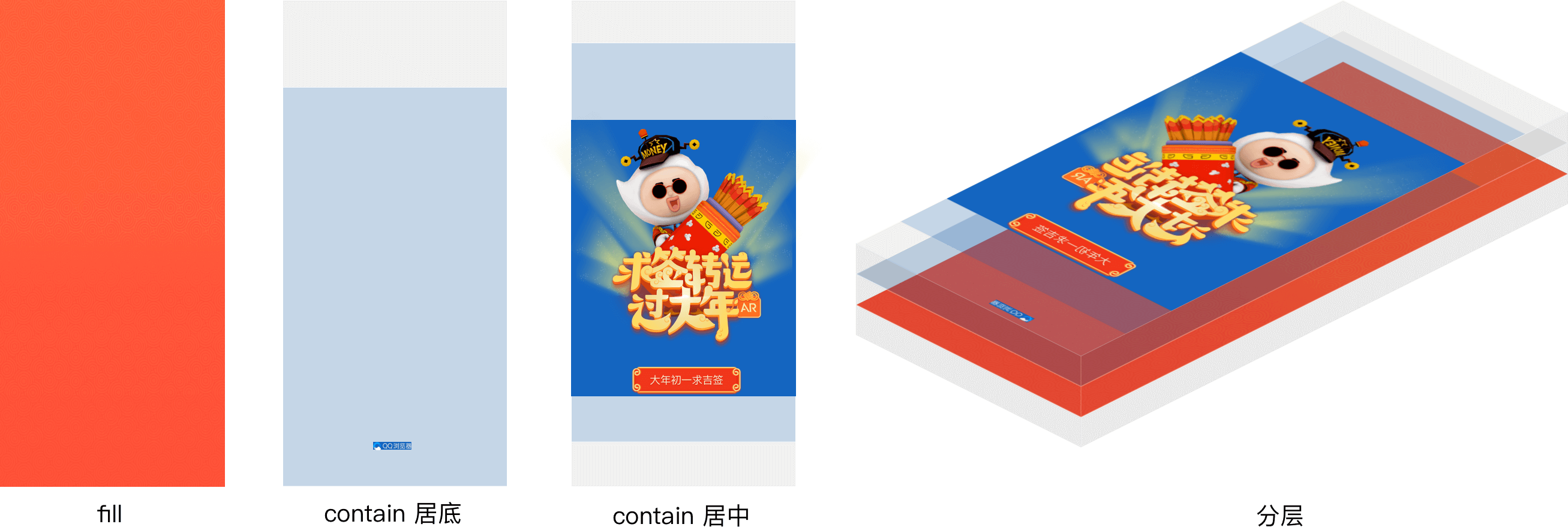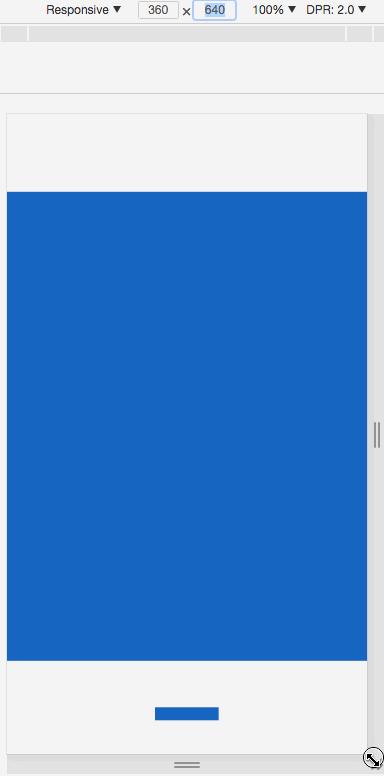# H5分层屏幕适配

## 屏幕适配## 优选 CSS

• 页面加载后 js 往往需要延时至少 70ms 才能获取正确的 webview 宽高
• css 往往最先执行，且 cssom 的解析往往和 dom 在最开始并行构建
• js 会等待 dom 和 cssom 处理完后才能执行，而 css 只需等待 dom
• 相比 js 在切换横竖屏时要切换 2 次进程来重绘，css 无需切换

## 整层适配

``````<!doctype html>
<html>
<body>
<style>
.layer {
position: absolute;
top: 0;
left: 0;
width: 100%;
height: 100%;
}
</style>
<!-- fill -->
<svg class="layer" viewBox="0 0 1080 1920" preserveAspectRatio="none"> <!-- 容器 -->
<rect x="0" y="0" width="1080" height="1920" fill="rgba(96,96,96,.08)"/>  <!-- 元素 -->
</svg>
<!-- contain 居中 -->
<svg class="layer" viewBox="0 0 1080 1920" preserveAspectRatio="xMidYMid meet"> <!-- 容器 -->
<rect x="0" y="233" width="1080" height="1407" fill="#1565C0"/>  <!-- 元素 -->
</svg>
<!-- contain 居底 -->
<svg class="layer" viewBox="0 0 1080 1920" preserveAspectRatio="xMidYMax meet"> <!-- 容器 -->
<rect x="444" y="1779" width="191" height="39" fill="#1565C0"/>  <!-- 元素 -->
</svg>
</body>
</html>
``````• 容器元素 应用 css 动画会造成频繁重排和重绘，导致卡顿。
• 将和 适配区 尺寸相同的 容器 提升为合成层时所占内存过大，有多少层内存就翻多少倍。

## 精简适配### 公式

``````设计稿
宽 v
高 g

横坐标 x
纵坐标 y
宽 w
高 h

横坐标 x3 = x*u/v
纵坐标 y3 = y*f/g

横坐标 x4 = m*u + (x - m*v)/w*w1 = m*v/w*w3 + (x - m*v)/w*w1
纵坐标 y4 = n*f + (y - n*g)/h*h1 = n*g/h*h3 + (y - n*g)/h*h1
宽 w3 = (w/v)*u
高 h3 = (h/g)*f
当 contain 方式适配时
缩放值 s = Math.min(f/g, u/v)
横向左留白占总留白 o = (m*v - x)/w
纵向上留白占总留白 p =  (n*g - y)/h
当 cover 方式适配时
缩放值 s = Math.max(f/g, u/v)
横向左超出占总超出 o = (x - m*v)/w
纵向上超出占总超出 p =  (y - n*g)/h

垂直居顶时 m = 0
垂直居中时 m = .5
垂直居底时 m = 1
水平居左时 n = 0
水平居中时 n = .5
水平居右时 n = 1

``````

### 实现示例

• 当设 max-width 为 `w/v`，max-height 为 `h/g` 时对应 contain 适配。
• 当设 min-width 为 `w/v`，min-height 为 `h/g` 时对应 cover 适配。
• 当设 width 为 `w/v`，height 为 `h/g` 时表示 fill 适配。
• contain 适配时，如果图片原始尺寸小于 `max-width``max-height` 时，用 `zoom: 10` 放大或者直接修改图片原始尺寸。
• cover 适配时，如果图片原始尺寸大于 `min-width``min-height` 时，用 `zoom: .1` 缩小或者直接修改图片原始尺寸。
• `top` `left` 中百分比是相对屏幕宽 `u` 和高 `f` 的，对应 `m*u``n*f`
• `transform` 中百分比是相对适配后元素宽 `w1` 和高 `h1` 的，对应 `(m*v + x)/w*w1 和 (n*f + y)/h*h1`
``````<!doctype html>
<html>
<body>
<style>
img {
/* min-width 和 min-height 构成了虚拟的容器 */
min-width: 50.37037037037037%; /* w3 = (w/v)*u 其中 w = 544，v = 1080 */
min-height: 7.395833333333333%; /* h3 = (h/g)*f 其中 h = 142，g = 1920 */
zoom: .1;
/* x4 = m*u + (x - m*v)/w*w1 */
/* y4 = n*f + (y - n*g)/h*h1 */
position: absolute;
left: 50%; /* m*u 其中 m = .5*/
top: 50%; /* n*f 其中 n = .5 */
transform:
translateX(-48.34558823529412%) /* (x - m*v)/w*w1 其中 x = 277，m = .5，v = 1080，w = 544 */
translateY(378.8732394366197%); /* (y - n*g)/h*h1 其中 y = 1498，n = .5，g = 1920，h = 142 */
}
</style>
<img src="http://ui.qzone.com/544x142"/> <!-- 元素 -->
</body>
</html>
``````

### background 实现示例

• `background-size` 值为 `contain` 时对应 contain 适配。
• `background-size` 值为 `cover` 时对应 cover 适配。
• `background-size` 值为 `100% 100%` 时对应 `fill 适配。
• `background-position` 百分比和 `o` `p` 意义相同
``````<!doctype html>
<html>
<body>
<style>
div {
position: absolute;
width: 50.37037037037037%; /* w3 = w/v*u 其中 w = 544，v = 1080 */
height: 7.395833333333333%; /* h3 = h/g*f 其中 h = 142，g = 1920 */
background: url(http://ui.qzone.com/544x142) no-repeat; /* 背景图做元素 */
background-size: cover;
left: 25.64814814814815%; /* x3 = x/v*u 其中 x = 277， v = 1080 */
top: 78.02083333333333%; /* y3 = y/g*f 其中 y = 1498， g = 1920 */
background-position-x: -48.34558823529412%; /* o = (x - m*v)/w 其中 m = .5 ， v = 1080，x = 277，w = 544*/
background-position-y: 378.8732394366197%; /* p = (y - n*g)/h 其中 n = .5 ， g = 1920，y = 1498，h = 142*/
}
</style>
<div></div> <!-- 容器 -->
</body>
</html>
``````

### 实现示例

• `preserveAspectRatio``meetOrSlice``meet` 时对应 contain 适配。
• `preserveAspectRatio``meetOrSlice``slice` 时对应 cover 适配。
• `preserveAspectRatio` 值为 `none` 时对应 fill 适配。
• 这里 `preserveAspectRatio``meetOrSlice` 相对的是容器，不是 适配区 这里用 `transform` 来定位，而 `preserveAspectRatio``meetOrSlice` 固定为 `xMinYMin`
``````<!doctype html>
<html>
<body>
<style>
svg {
position: absolute;
width: 50.37037037037037%;
height: 7.395833333333333%;
/* x4 = m*v/w*w3 + (x - m*v)/w*w1 */
/* y4 = n*g/h*h3 + (y - n*g)/h*h1 */
top: 0;
left: 0;
transform:
translateX(99.26470588235294%) /* m*v/w*w3 其中 m = .5，v = 1080，w = 544 */
translateY(676.056338028169%); /* n*g/h*h3 其中 n = .5，g = 1920，h = 142 */
overflow: visible;
}
svg image {
transform:
translateX(-48.34558823529412%) /* (x - m*v)/w*w1 其中 x = 277，m = .5，v = 1080，w = 544 */
translateY(378.8732394366197%); /* (y - n*g)/h*h1 其中 y = 1498，n = .5，g = 1920，h = 142 */
}
</style>
<svg viewBox="0 0 544 142" preserveAspectRatio="xMinYMin meet"> <!-- 容器 -->
<image width="544" height="142" xlink:href="http://ui.qzone.com/544x142"/> <!-- 元素 -->
</svg>
</body>
</html>
``````

### 文字处理

• 文字固定或单行不固定，`svg``text` 标签可以处理
• 文字固定或单行不固定还可以将文字转为图片
• 文字多行不固定，可以借助 `svg``foreignObject` 嵌入普通 `div`